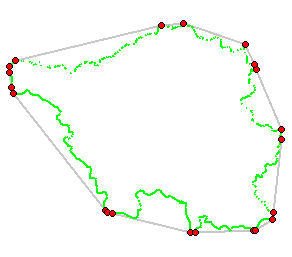CGAL 4.12.1 - 2D Convex Hulls and Extreme Points
User Manual

# Introduction

A subset $$S \subseteq \mathbb{R}^2$$ is convex if for any two points $$p$$ and $$q$$ in the set the line segment with endpoints $$p$$ and $$q$$ is contained in $$S$$. The convex hull of a set $$S$$ is the smallest convex set containing $$S$$. The convex hull of a set of points $$P$$ is a convex polygon with vertices in $$P$$. A point in $$P$$ is an extreme point (with respect to $$P$$) if it is a vertex of the convex hull of $$P$$. A set of points is said to be strongly convex if it consists of only extreme points.

This chapter describes the functions provided in CGAL for producing convex hulls in two dimensions as well as functions for checking if sets of points are strongly convex are not. There are also a number of functions described for computing particular extreme points and subsequences of hull points, such as the lower and upper hull of a set of points.# Convex Hull

CGAL provides implementations of several classical algorithms for computing the counterclockwise sequence of extreme points for a set of points in two dimensions (i.e., the counterclockwise sequence of points on the convex hull). The algorithms have different asymptotic running times and require slightly different sets of geometric primitives. Thus you may choose the algorithm that best fits your setting.

Each of the convex hull functions presents the same interface to the user. That is, the user provides a pair of iterators, first and beyond, an output iterator result, and a traits class traits. The points in the range [first, beyond) define the input points whose convex hull is to be computed. The counterclockwise sequence of extreme points is written to the sequence starting at position result, and the past-the-end iterator for the resulting set of points is returned. The traits classes for the functions specify the types of the input points and the geometric primitives that are required by the algorithms. All functions provide an interface in which this class need not be specified and defaults to types and operations defined in the kernel in which the input point type is defined.

Given a sequence of $$n$$ input points with $$h$$ extreme points, the function convex_hull_2() uses either the output-sensitive $$O(n h)$$ algorithm of Bykat  (a non-recursive version of the quickhull  algorithm) or the algorithm of Akl and Toussaint, which requires $$O(n \log n)$$ time in the worst case. The algorithm chosen depends on the kind of iterator used to specify the input points. These two algorithms are also available via the functions ch_bykat() and ch_akl_toussaint(), respectively. Also available are the $$O(n \log n)$$ Graham-Andrew scan algorithm ,  (ch_graham_andrew()), the $$O(n h)$$ Jarvis march algorithm  (ch_jarvis()), and Eddy's $$O(n h)$$ algorithm  (ch_eddy()), which corresponds to the two-dimensional version of the quickhull algorithm. The linear-time algorithm of Melkman for producing the convex hull of simple polygonal chains (or polygons) is available through the function ch_melkman().

# Example using Graham-Andrew's Algorithm

In the following example a convex hull is constructed from point data read from standard input using Graham_Andrew algorithm. The resulting convex polygon is shown at the standard output console. The same results could be achieved by substituting the function ch_graham_andrew() by other function like ch_bykat().

#include <CGAL/Exact_predicates_inexact_constructions_kernel.h>
#include <CGAL/ch_graham_andrew.h>
typedef K::Point_2 Point_2;
int main()
{
std::istream_iterator< Point_2 > in_start( std::cin );
std::istream_iterator< Point_2 > in_end;
std::ostream_iterator< Point_2 > out( std::cout, "\n" );
CGAL::ch_graham_andrew( in_start, in_end, out );
return 0;
}

# Extreme Points and Hull Subsequences

In addition to the functions for producing convex hulls, there are a number of functions for computing sets and sequences of points related to the convex hull.

The functions lower_hull_points_2() and upper_hull_points_2() provide the computation of the counterclockwise sequence of extreme points on the lower hull and upper hull, respectively. The algorithm used in these functions is Andrew's variant of Graham's scan algorithm , , which has worst-case running time of $$O(n \log n)$$.

There are also functions available for computing certain subsequences of the sequence of extreme points on the convex hull. The function ch_jarvis_march() generates the counterclockwise ordered subsequence of extreme points between a given pair of points and ch_graham_andrew_scan() computes the sorted sequence of extreme points that are not left of the line defined by the first and last input points.

Finally, a set of functions (ch_nswe_point(), ch_ns_point(), ch_we_point(), ch_n_point(), ch_s_point(), ch_w_point(), ch_e_point()) is provided for computing extreme points of a 2D point set in the coordinate directions.

# Traits Classes

Each of the functions used to compute convex hulls or extreme points is parameterized by a traits class, which specifies the types and geometric primitives to be used in the computation. There are several implementations of 2D traits classes provided in the library. The class Convex_hull_traits_2 corresponds to the default traits class that provides the types and predicates presented in the 2-dimensional CGAL kernel in which the input points lie. The class Convex_hull_constructive_traits_2 is a second traits class based on CGAL primitives but differs from Convex_hull_traits_2 in that some of its primitives reuse intermediate results to speed up computation.

In addition, the 2D and 3D Linear Geometric Kernel provides three projective traits classes (Projection_traits_xy_3, Projection_traits_xz_3, and Projection_traits_yz_3), which may be used to compute the convex hull of a set of three-dimensional points projected into each of the three coordinate planes.

# Convexity Checking

The functions is_ccw_strongly_convex_2() and is_cw_strongly_convex_2() check whether a given sequence of 2D points forms a (counter)clockwise strongly convex polygon. These are used in postcondition testing of the two-dimensional convex hull functions.